Courses

# Surveying (Part - 8) Civil Engineering (CE) Notes | EduRev

## Civil Engineering (CE) : Surveying (Part - 8) Civil Engineering (CE) Notes | EduRev

The document Surveying (Part - 8) Civil Engineering (CE) Notes | EduRev is a part of the Civil Engineering (CE) Course Civil Engineering SSC JE (Technical).
All you need of Civil Engineering (CE) at this link: Civil Engineering (CE)

Surveying (Part 8)

Tangential Method of Tacheometry: In tangential Tacheometery, horizontal and vertical distance from the instrument to the staff position, are computed from the observed vertical angle of two targets fixed at a known distance S on the staff.

DEPENDING UPON THE VERTICAL ANGLES, THREE CASE MAY BE ARISE :

1. Both vertical angles may be elevation angles.
2. Both vertical angle may be depression angles.
3. One of the angle may be an elevation angle and the other may be a depression angle.

Case 1 : Distance and elevation angle :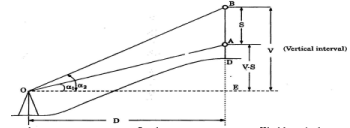Let A and B represent two targets fixed S metres apart on a staff held vertical at D.
Let O represent the trunnion axis of the theodolite.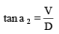þ V = D tan a2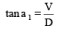þ V - S = D tan a1

Substracting equation (ii) from equation (i), we get S = D (tan a2 – tan a1)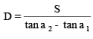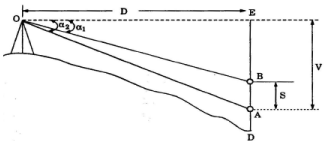CASE 2. Distance and depression angle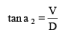þ  V = D tana 2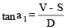þ V - S = D tana 1

Substracting equation (ii) from equation (i), we get

S = D (tan a2– tana1)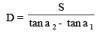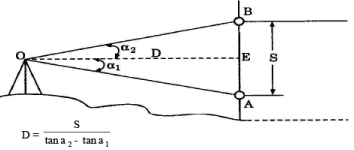Note : The most common method of tacheometry is a fixed hair stadia method, using a staff held vertically.

THE SUBSTANCE BAR : The substance bar is an instrument usd for measuring horizontal distances in the area where direct chaining becomes difficult due to undulations or other obstructions.
Comput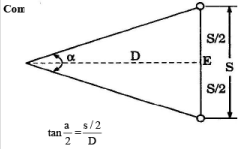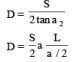(where a is very small)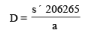(where a is very small)

Offer running on EduRev: Apply code STAYHOME200 to get INR 200 off on our premium plan EduRev Infinity!

## Civil Engineering SSC JE (Technical)

109 docs|50 tests

,

,

,

,

,

,

,

,

,

,

,

,

,

,

,

,

,

,

,

,

,

;